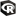# Generic R

This category contains 6 nodes.

##### Convert to R

Takes a data table as input and pushes it to R

##### Convert to Table

Takes a R workspace as input and extracts a data frame as KNIME table.

##### Open in R

Opens R session with R workspace from KNIME.

##### R Plot

A generic R plot node that takes an R workspace input and returns figure created by R

##### R Snippet

A generic R scripting node that takes an R workspace input and returns the altered R workspace as output

##### R Source

A generic R scripting node that creates a fresh R workspace as output